# Lighting Properties#

Control aspects of the rendered mesh’s lighting such as Ambient, Diffuse, and Specular. These options only work if the `lighting` argument to `add_mesh` is `True` (it’s `True` by default).

You can turn off all lighting for the given mesh by passing `lighting=False` to `add_mesh`.

```import pyvista as pv
from pyvista import examples

cpos = [(575848.0, 5128459.0, 22289.0), (562835.0, 5114981.5, 2294.5), (-0.5, -0.5, 0.7)]
```

First, lets take a look at the mesh with default lighting conditions

```mesh.plot(cpos=cpos, show_scalar_bar=False)
``````mesh.plot(lighting=False, cpos=cpos, show_scalar_bar=False)
```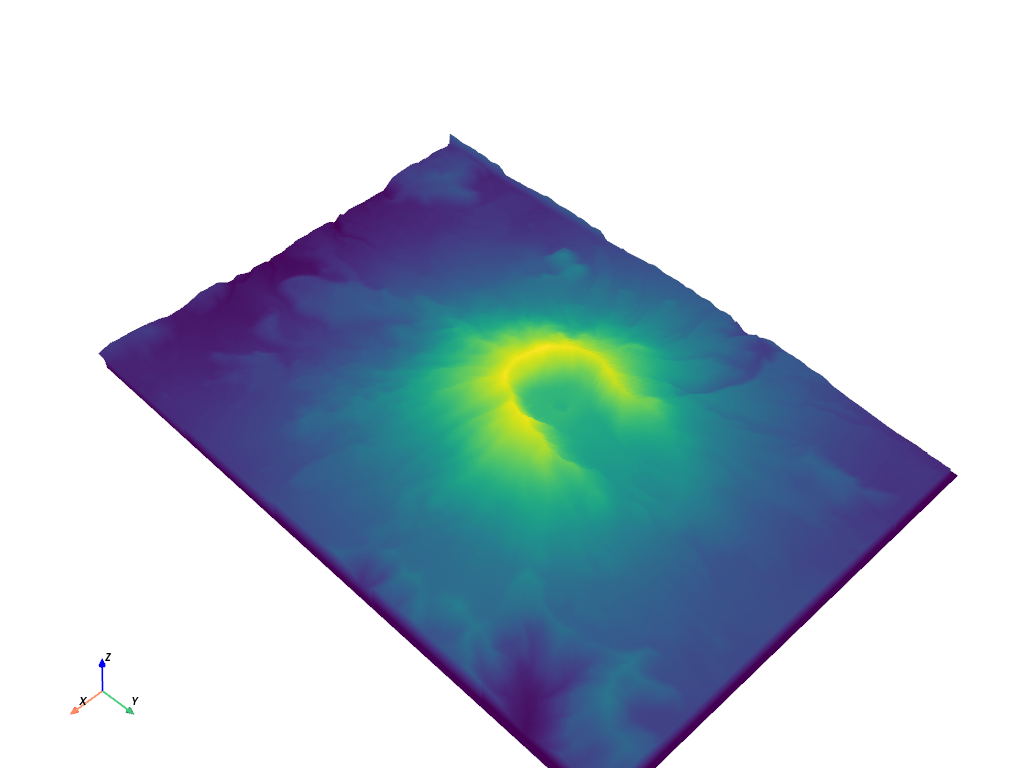Demonstration of the specular property

```p = pv.Plotter(shape=(1, 2), window_size=[1500, 500])

p.subplot(0, 0)

p.subplot(0, 1)
s = 1.0

p.view_isometric()
p.show(cpos=cpos)
```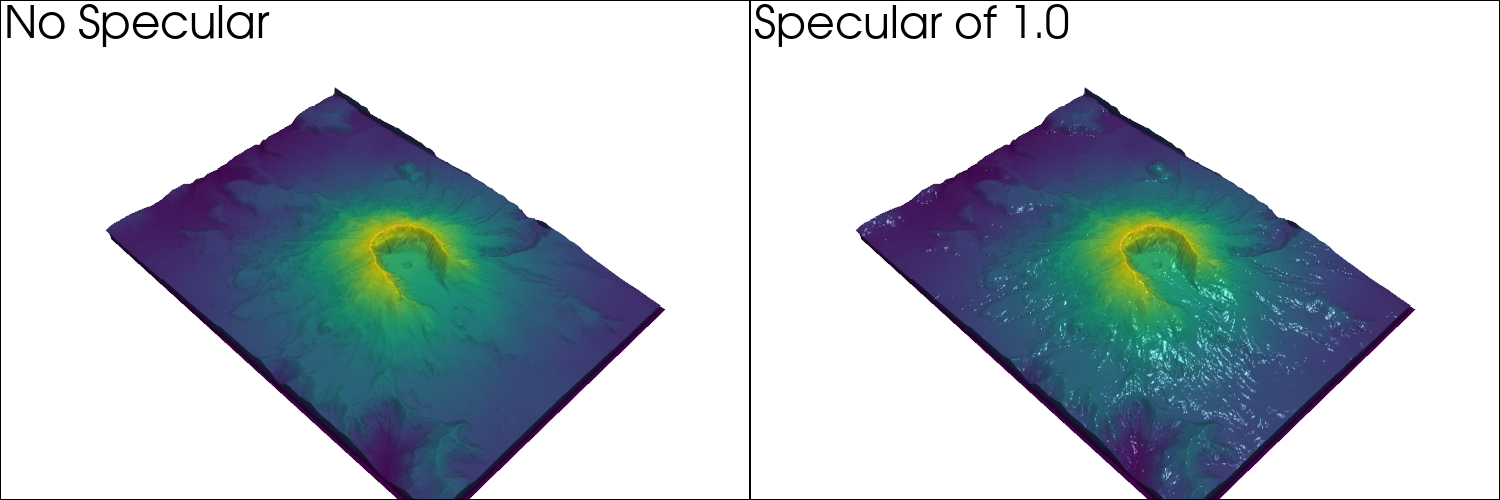Just specular

```mesh.plot(specular=0.5, cpos=cpos, show_scalar_bar=False)
```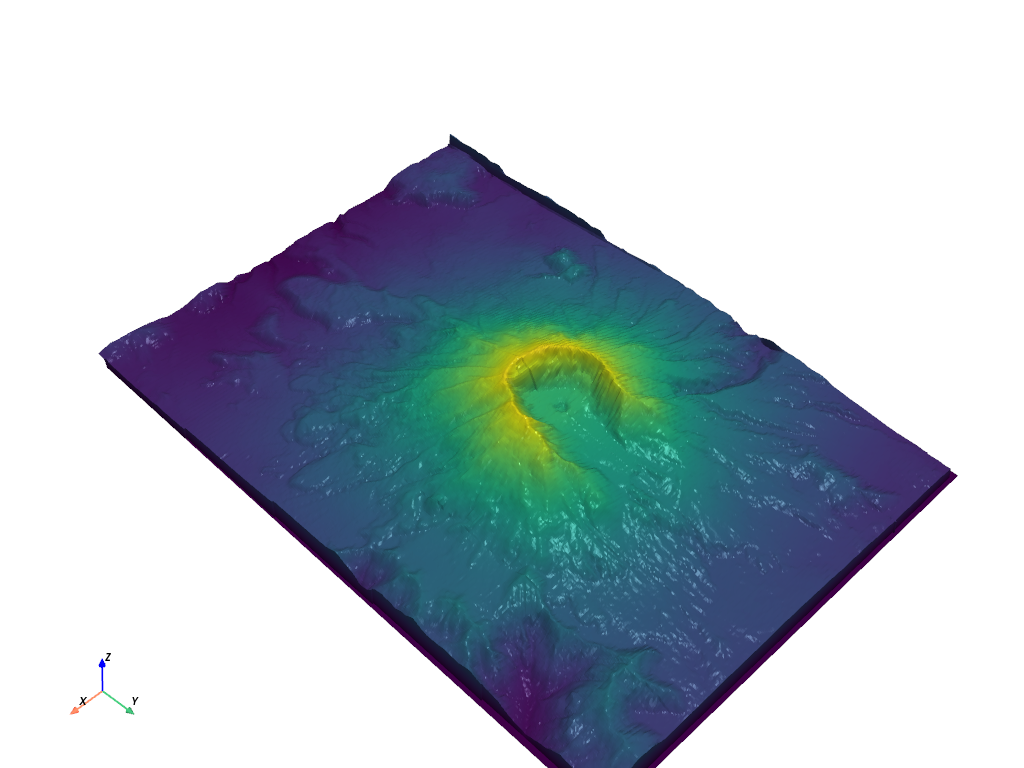Specular power

```mesh.plot(specular=0.5, specular_power=15, cpos=cpos, show_scalar_bar=False)
```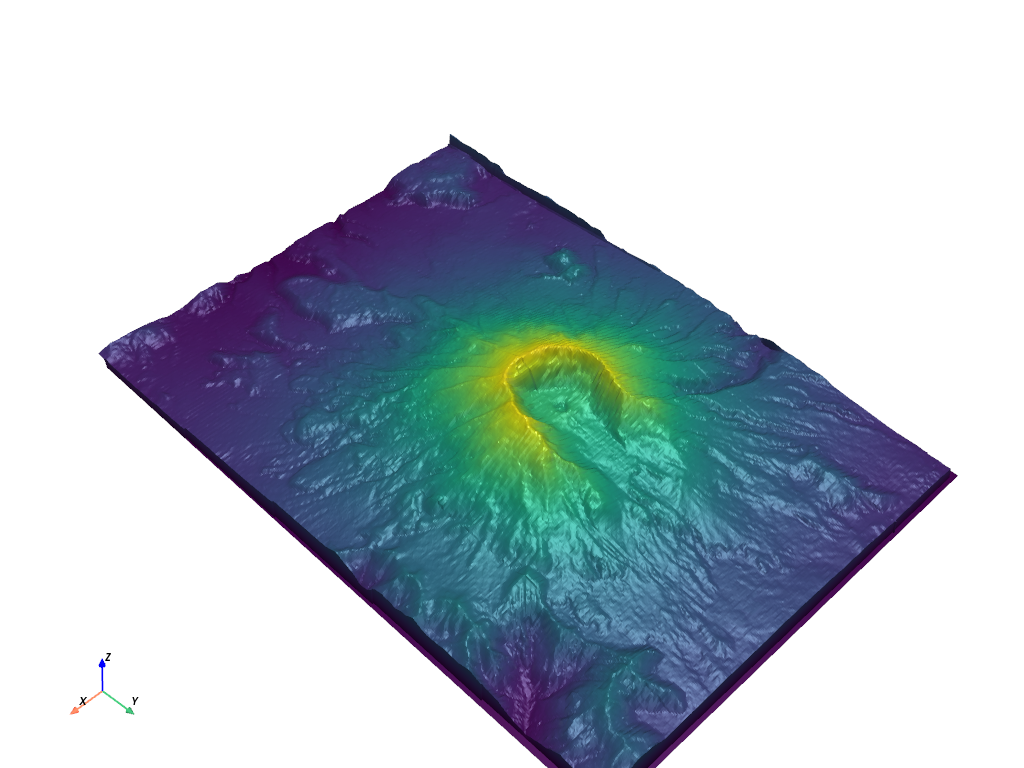Demonstration of all three in use

```mesh.plot(diffuse=0.5, specular=0.5, ambient=0.5, cpos=cpos, show_scalar_bar=False)
```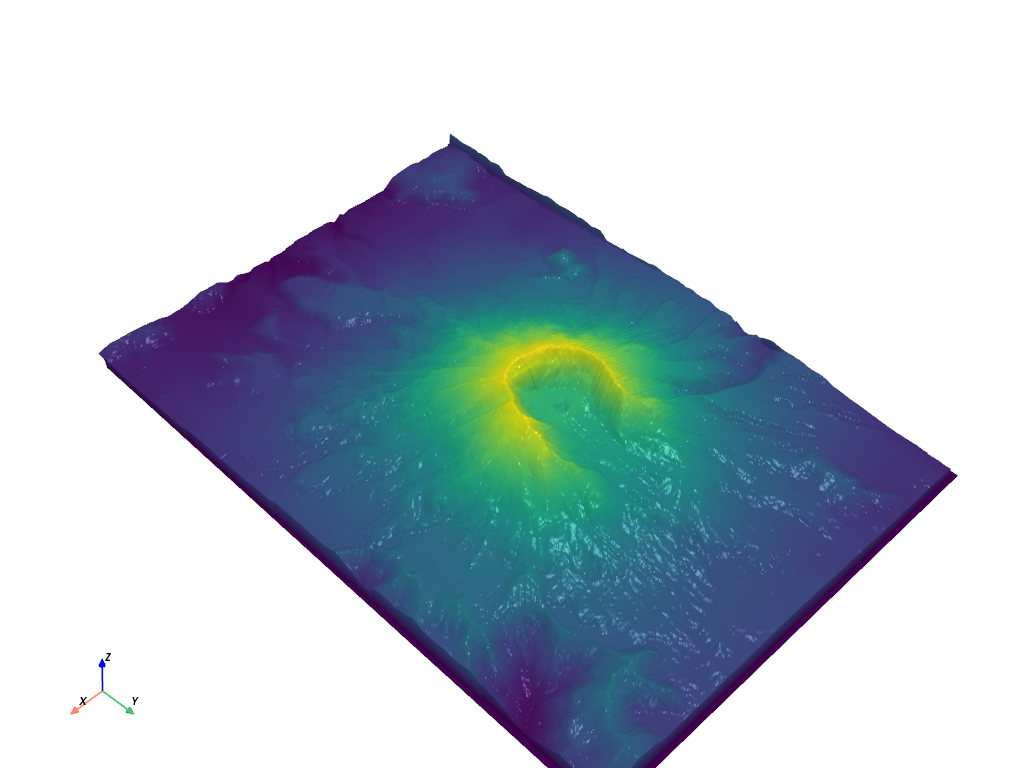For detailed control over lighting conditions in general see the Lighting examples.

Total running time of the script: ( 0 minutes 3.294 seconds)

Gallery generated by Sphinx-Gallery